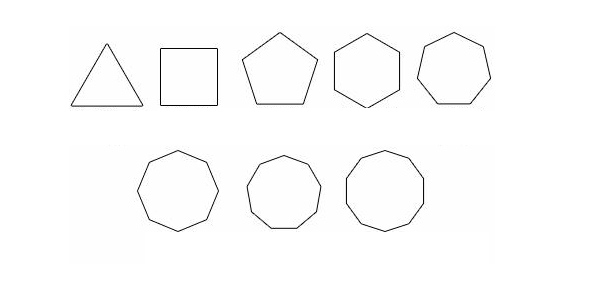# Geometry Polygons Quiz

10 QuestionsSettings.

Related Topics
• 1.
How many sides does a pentagon have?
• A.

Three

• B.

Four

• C.

Five

• D.

Six

• 2.
What is the name of a four sided polygon?
• A.

Triangle

• B.

Octagon

• C.

Pentagon

• D.

• 3.
What is the least number of sides a polygon can have?
• A.

Two

• B.

Three

• C.

Four

• D.

Five

• 4.
How many angles are there in a triangle?
• A.

Three

• B.

Five

• C.

Six

• D.

Ten

• 5.
A stop sign is what type of polygon?
• A.

• B.

Octagon

• C.

Hexagon

• D.

Pentagon

• 6.
Can a polygon have cruved sides?
• A.

Yes

• B.

No

• 7.
What makes a polygon a regular polygon?
• A.

All sides are the same lenght

• B.

All angles are congruent

• C.

All angles are 90 degrees

• D.

All angles and sides are the same

• 8.
Which of the following is NOT a polygon?
• A.

Square

• B.

Pentagon

• C.

Circle

• D.

Triangle

• 9.
What type of polygon is this?
• A.

• B.

Pentagon

• C.

Octagon

• D.

Heptagon

• 10.
Is this polygon a regular polygon?
• A.

Yes

• B.

No